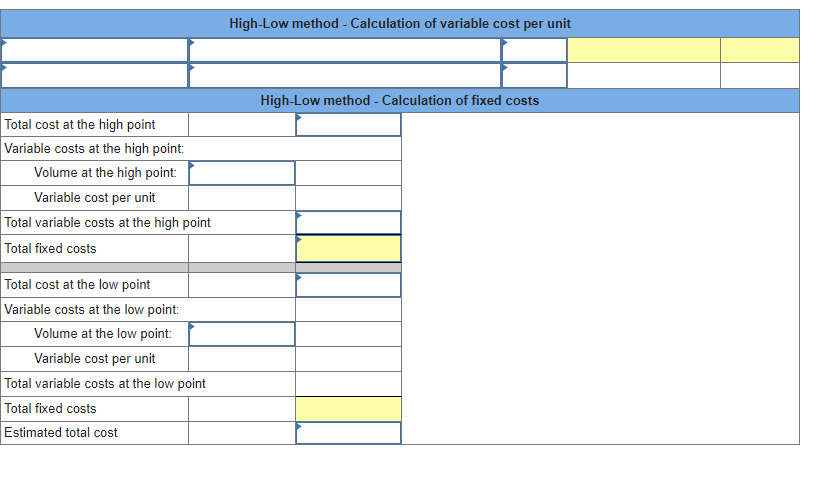# Exercise 21-6 Cost behavior estimation-scatter diagram and high-low LO P1 Felix & Co. reports the following...

###### Question:Exercise 21-6 Cost behavior estimation-scatter diagram and high-low LO P1 Felix & Co. reports the following information about its units produced and total costs. Units Produced Period 1 2 3 4 5 410 810 1,210 1,610 Total Costs $2,510 3,110 3,710 4,310 4,910 Period 6 7 8 9 Units Produced 2,010 2,410 2,810 3,210 3,610 Total Costs$5,510 6,110 6,710 7,310 8,286 LO Estimate total costs if 3,010 units are produced. Complete the below table to calculate the fixed cost and variable cost of sale using the high-low method. High-Low method - Calculation of variable cost per unit High-Low method - Calculation of fixed costs Total cost at the int Variable costs at the high point:
High-Low method - Calculation of variable cost per unit High-Low method - Calculation of fixed costs Total cost at the high point Variable costs at the high point: Volume at the high point Variable cost per unit Total variable costs at the high point Total fixed costs Total cost at the low point Variable costs at the low point: Volume at the low point Variable cost per unit Total variable costs at the low point Total fixed costs Estimated total cost